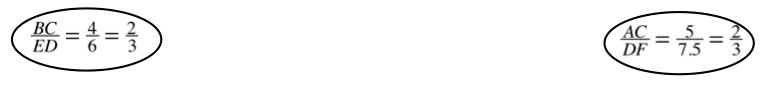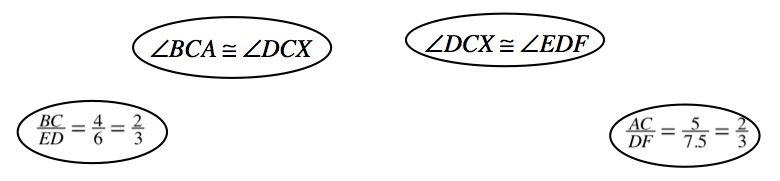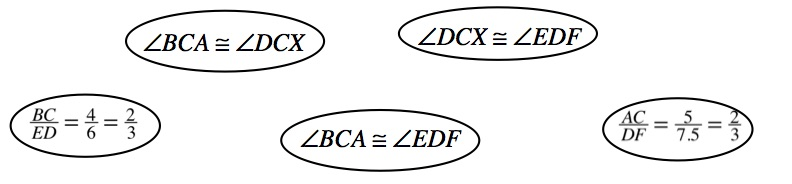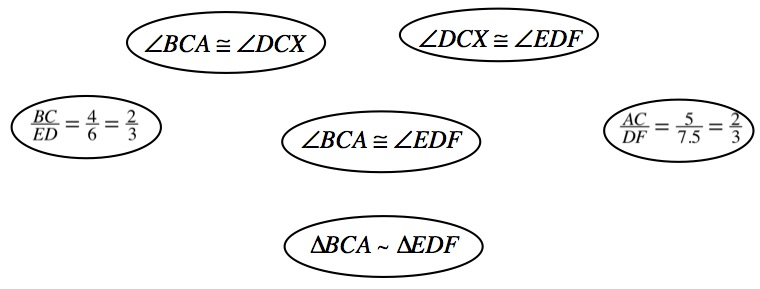### Home > CCG > Chapter 5 > Lesson 5.1.2 > Problem5-23

5-23.

Examine the triangles below. Are the triangles similar? If so, show how you know with a flowchart. If not, explain how you know they cannot be similar.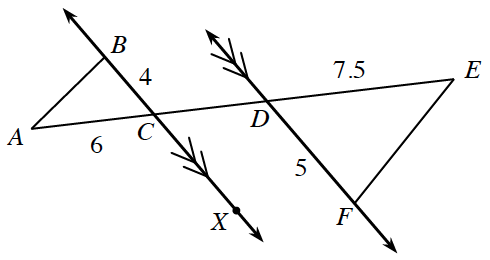Be sure to add reasons and arrows to the flowchart. Bubble: B, C divided by A, C equals 4 sixths = 2 thirds and Bubble: D, F divided by D, E equals 5 divided by 7.5 = 2 thirds.

Two more bubbles placed above and between the first two: Bubble: angle B, C, A is congruent to angle D, C, X and Bubble: angle D, C, X is congruent to angle E, D, F

Another bubble placed between the first two bubbles: angle B, C, A is congruent to angle E, D, F

Another bubble placed below the last bubble: Triangle B, C, A is similar to triangle E, D, F.